Anzeige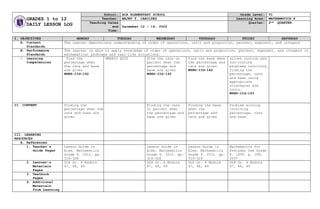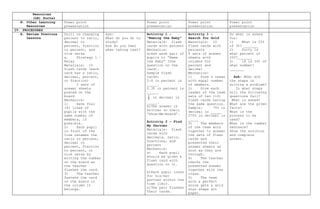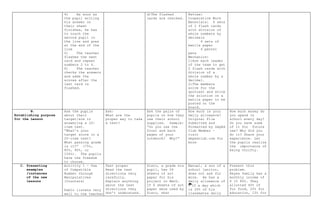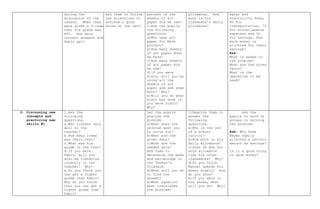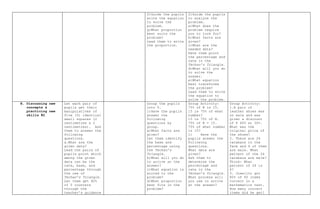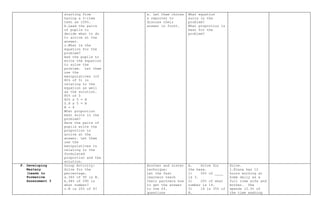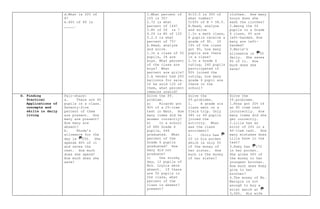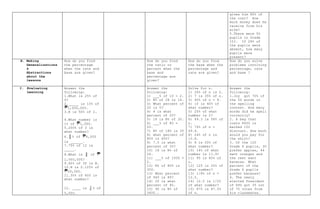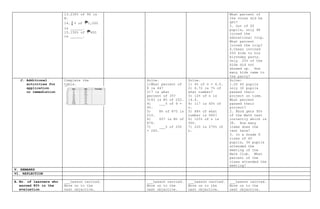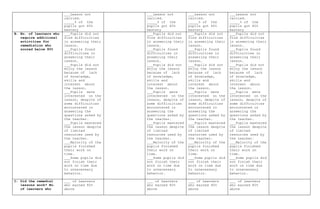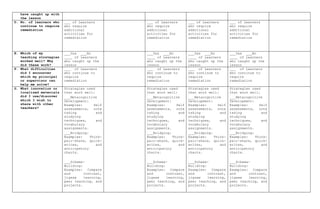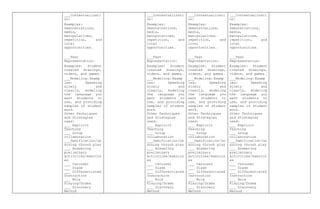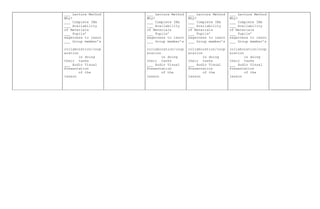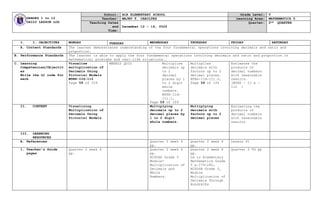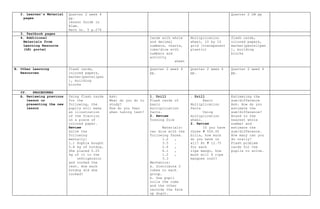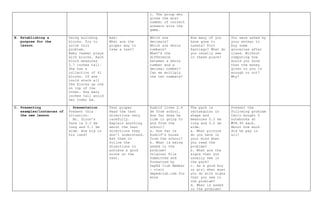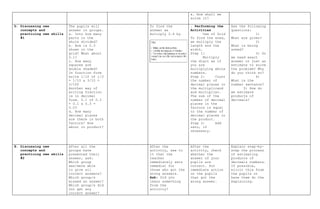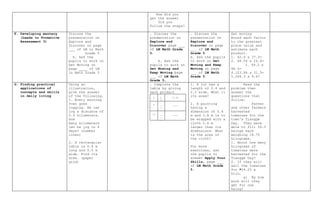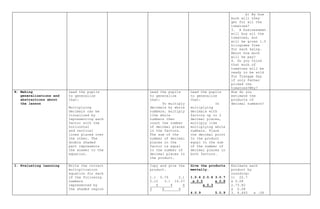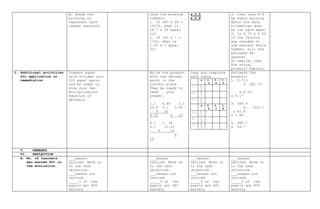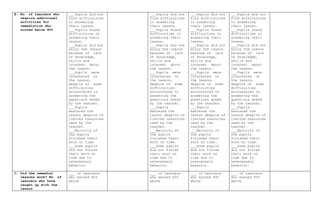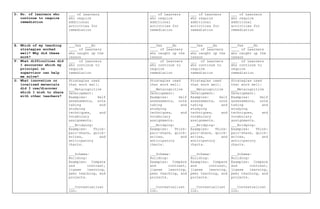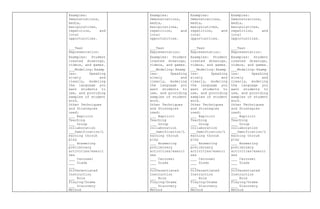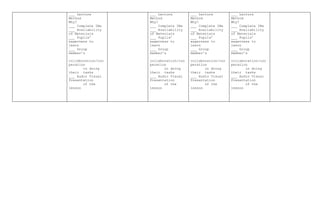1 von 24
Anzeige

1. GRADES 1 to 12 DAILY LESSON LOG School: ACA ELEMENTARY SCHOOL Grade Level: VI Teacher: MAJEV T. CABILTES Learning Area: MATHEMATICS 6 Teaching Dates and Time: December 12 – 16, 2022 Quarter: 2nd QUARTER I. OBJECTIVES MONDAY TUESDAY WEDNESDAY THURSDAY FRIDAY SATURDAY A. Content Standards The learner demonstrate understanding of order of operations, ratio and proportion, percent, exponent, and integers B. Performance Standards The learner is able to apply knowledge of order of operations, ratio and proportion, percent, exponent, and integers in mathematical problems and real-life situations. C. Learning Competencies Find the percentage when the rate and base are given M6NS-IId-142 WEEKLY QUIZ Find the rate or percent when the percentage and base are given M6NS-IId-142 Find the base when the percentage and rate are given M6NS-IId-142 solves routine and non-routine problems involving finding the percentage, rate and base using appropriate strategies and tools. M6NS-IId-143 II. CONTENT Finding the percentage when the rate and base are given Finding the rate or percent when the percentage and base are given Finding the base when the percentage and rate are given Problem solving involving percentage, rate and base III. LEARNING RESOURCES A. References 1. Teacher’s Guide Pages Lesson Guide in Elem. Mathematics Grade 6. 2012. pp. 316-328 Lesson Guide in Elem. Mathematics Grade 6. 2012. pp. 316-328 Lesson Guide in Elem. Mathematics Grade 6. 2012. pp. 316-328 Mathematics for Everyday Use Grade 6. 1999. p. 199, 203* 2. Learner’s Materials Pages DLP Gr. 6 Module 47, 48, 49 DLP Gr. 6 Module 47, 48, 49 DLP Gr. 6 Module 47, 48, 49 DLP Gr. 6 Module 47, 48, 49 3. Textbook Pages 4. Additional Materials from Learning
6. starting from having a 5-item test as 100%. b.Lead the pairs of pupils to decide what to do to arrive at the answer. c.What is the equation for the problem? Ask the pupils to write the equation to solve the problem. Let them use the manipulatives (of 80% of 5) in relating to the equation as well as the solution. 80% of 5 80% x 5 = N 0.8 x 5 = N N = 4 What proportion best suits in the problem? Have the pairs of pupils write the proportion to arrive at the answer. Let them use the manipulatives in relating to the formulated proportion and the solution. e. Let them choose a reporter to discuss their answer in front. What equation suits in the problem? What proportion is best for the problem? F. Developing Mastery (Leads to Formative Assessment 3 Group Activity: Solve for the percentage. a.36% of 95 is N. b.48% of 290 is what number? c.N is 20% of 8? Brother and sister technique: Let the fast learners teach their partners how to get the answer to the ff. questions A. Solve for the base. 1) 50% of ____ is 3. 2) 20% of what number is 14. 3) 14 is 35% of N. Solve. 1.Elena has 12 hours working at home daily as a full time wife and mother. She spends 12.5% of the time washing
8. gives him 80% of the cost? How much money does he receive from his wife? 5.There were 50 pupils in Grade III. If 28% of the pupils were absent, how many pupils were present? H. Making Generalizations & Abstractions about the lessons How do you find the percentage when the rate and base are given? How do you find the ratio or percent when the base and percentage are given? How do you find the base when the percentage and rate are given? How do you solve problems involving percentage, rate and base ? I. Evaluating Learning Answer the following: 1.What is 25% of 4? 2._____ is 10% of 1,000,000. 3.N is 50% of 2. 4.What number is 1% of 1,000. 5.200% of 3 is what number? 6. % of 6,000 is ______. 7.75% of 12 is _____. 8.What is of 1,000,000? 9.60% of 30 is N. 10.N is 0.125% of 20,000. 11.30% of 600 is what number? 12. ____ is % of 5,000. Answer the following: 1) ___% of 10 = 2. 2) N% of 28 is 14. 3) What percent of 20 is 5? 4) 4 is what percent of 20? 5) 18 is N% of 30. 6) ___% of 80 = 20. 7) N% of 180 is 35 8) what percent of 800 is 400? 9) 7.5 is what percent of 30? 10) 18 is N% of 24. 11) ___% of 1000 = 2. 12) N% of 800 is 300. 13) What percent of 940 is 89? 14) 20 is what percent of 40. 15) 90 is N% of 3600.. Solve for n. 1) 20% of n is 2. 2) 7 is 35% of n. 3) 40% of n = 8. 4) 10 is 40% of what number? 5) 25% of what number is 2? 6) 49.3 is 58% of n. 7) 76% of n = 49.4. 8) 24% of n is 10.8. 9) 8 is 20% of what number? 10) 14% of what number is 11.9? 11) 95 is 80% of n. 12) 125 is 20% of what number? 13) 1/8% of n = 12.5. 14) 12.5 is 1/2% of what number? 15) 875 is 87.5% of n. Answer the following: 1.Joy got 70% of the 50 words in the spelling contest. How many words did he spell correctly? 2. A bag that costs P450 is marked 15% discount. How much would you pay for the shirt? 3. Of the 120 Grade 6 pupils, 30 prefer apples, 44 want oranges and the rest want bananas. What percent of the Grade 6 pupils prefer bananas? 4. The newly elected President of SPG got 35 out of 70 votes from his classmates. 8 5 8 3 2 1
9. 13.230% of 90 is N. 14. % of 1,000 is ______. 15.150% of 400 is ______. What percent of the votes did he get? 5. Out of 50 pupils, only 48 joined the educational trip. What percent joined the trip? 6.Cesar invited 250 kids to his birthday party. Only 25% of the kids did not showed up. How many kids came to the party? J. Additional activities for application or remediation Complete the table. Solve. 1)What percent of 8 is 64? 2)7 is what percent of 35? 3)63 is N% of 252. 4) ___% of 9 = 45. 5) N% of 875 is 210. 6) 657 is N% of 876. 7) ___% of 250 = 245. Solve. 1) 6% of n = 4.5. 2) 6.72 is 7% of what number? 3) 12% of n is 14.4. 4) 117 is 65% of n. 5) 88% of what number is 660? 6) 125% of n is 900. 7) 220 is 275% of n. Solve: 1.Of 40 pupils only 30 pupils passed their project on time. What percent passed their project? 2. Rhod gets 95% of the Math test correctly which is 38. How many items does the test have? 3. In a Grade 6 class of 40 pupils, 36 pupils attended the meeting of the Math Club. What percent of the class attended the meeting? V. REMARKS VI. REFLECTION A. No. of learners who earned 80% in the evaluation ___Lesson carried. Move on to the next objective. ___Lesson carried. Move on to the next objective. ___Lesson carried. Move on to the next objective. ___Lesson carried. Move on to the next objective. 5 1
11. have caught up with the lesson D. No. of learners who continue to require remediation ___ of Learners who require additional activities for remediation ___ of Learners who require additional activities for remediation ___ of Learners who require additional activities for remediation ___ of Learners who require additional activities for remediation E. Which of my teaching strategies worked well? Why did these work? ___Yes ___No ____ of Learners who caught up the lesson ___Yes ___No ____ of Learners who caught up the lesson ___Yes ___No ____ of Learners who caught up the lesson ___Yes ___No ____ of Learners who caught up the lesson F. What difficulties did I encounter which my principal or supervisor can help me solve? ___ of Learners who continue to require remediation ___ of Learners who continue to require remediation ___ of Learners who continue to require remediation ___ of Learners who continue to require remediation G. What innovation or localized materials did I use/discover which I wish to share with other teachers? Strategies used that work well:  ___Metacognitive Development: Examples: Self assessments, note taking and studying techniques, and vocabulary assignments.  ___Bridging: Examples: Think- pair-share, quick- writes, and anticipatory charts.   ___Schema- Building: Examples: Compare and contrast, jigsaw learning, peer teaching, and projects.  Strategies used that work well:  ___Metacognitive Development: Examples: Self assessments, note taking and studying techniques, and vocabulary assignments.  ___Bridging: Examples: Think- pair-share, quick- writes, and anticipatory charts.   ___Schema- Building: Examples: Compare and contrast, jigsaw learning, peer teaching, and projects.  Strategies used that work well:  ___Metacognitive Development: Examples: Self assessments, note taking and studying techniques, and vocabulary assignments.  ___Bridging: Examples: Think- pair-share, quick- writes, and anticipatory charts.   ___Schema- Building: Examples: Compare and contrast, jigsaw learning, peer teaching, and projects.  Strategies used that work well:  ___Metacognitive Development: Examples: Self assessments, note taking and studying techniques, and vocabulary assignments.  ___Bridging: Examples: Think- pair-share, quick- writes, and anticipatory charts.   ___Schema- Building: Examples: Compare and contrast, jigsaw learning, peer teaching, and projects. 
12.  ___Contextualizati on:  Examples: Demonstrations, media, manipulatives, repetition, and local opportunities.   ___Text Representation:  Examples: Student created drawings, videos, and games.  ___Modeling: Examp les: Speaking slowly and clearly, modeling the language you want students to use, and providing samples of student work. Other Techniques and Strategies used: ___ Explicit Teaching ___ Group collaboration ___Gamification/Le arning throuh play ___ Answering preliminary activities/exercis es ___ Carousel ___ Diads ___ Differentiated Instruction ___ Role Playing/Drama ___ Discovery Method  ___Contextualizati on:  Examples: Demonstrations, media, manipulatives, repetition, and local opportunities.   ___Text Representation:  Examples: Student created drawings, videos, and games.  ___Modeling: Examp les: Speaking slowly and clearly, modeling the language you want students to use, and providing samples of student work. Other Techniques and Strategies used: ___ Explicit Teaching ___ Group collaboration ___Gamification/Le arning throuh play ___ Answering preliminary activities/exercis es ___ Carousel ___ Diads ___ Differentiated Instruction ___ Role Playing/Drama ___ Discovery Method  ___Contextualizati on:  Examples: Demonstrations, media, manipulatives, repetition, and local opportunities.   ___Text Representation:  Examples: Student created drawings, videos, and games.  ___Modeling: Examp les: Speaking slowly and clearly, modeling the language you want students to use, and providing samples of student work. Other Techniques and Strategies used: ___ Explicit Teaching ___ Group collaboration ___Gamification/Le arning throuh play ___ Answering preliminary activities/exercis es ___ Carousel ___ Diads ___ Differentiated Instruction ___ Role Playing/Drama ___ Discovery Method  ___Contextualizati on:  Examples: Demonstrations, media, manipulatives, repetition, and local opportunities.   ___Text Representation:  Examples: Student created drawings, videos, and games.  ___Modeling: Examp les: Speaking slowly and clearly, modeling the language you want students to use, and providing samples of student work. Other Techniques and Strategies used: ___ Explicit Teaching ___ Group collaboration ___Gamification/Le arning throuh play ___ Answering preliminary activities/exercis es ___ Carousel ___ Diads ___ Differentiated Instruction ___ Role Playing/Drama ___ Discovery Method
13. ___ Lecture Method Why? ___ Complete IMs ___ Availability of Materials ___ Pupils’ eagerness to learn ___ Group member’s collaboration/coop eration in doing their tasks ___ Audio Visual Presentation of the lesson ___ Lecture Method Why? ___ Complete IMs ___ Availability of Materials ___ Pupils’ eagerness to learn ___ Group member’s collaboration/coop eration in doing their tasks ___ Audio Visual Presentation of the lesson ___ Lecture Method Why? ___ Complete IMs ___ Availability of Materials ___ Pupils’ eagerness to learn ___ Group member’s collaboration/coop eration in doing their tasks ___ Audio Visual Presentation of the lesson ___ Lecture Method Why? ___ Complete IMs ___ Availability of Materials ___ Pupils’ eagerness to learn ___ Group member’s collaboration/coop eration in doing their tasks ___ Audio Visual Presentation of the lesson
14. GRADES 1 to 12 DAILY LESSON LOG School: ACA ELEMENTARY SCHOOL Grade Level: V Teacher: MAJEV T. CABILTES Learning Area: MATHEMATICS 5 Teaching Dates and Time: December 12 – 16, 2022 Quarter: 2nd QUARTER I. I. OBJECTIVES MONDAY  TUESDAY WEDNESDAY THURSDAY FRIDAY SATURDAY A. Content Standards The learner demonstrates understanding of the four fundamental operations involving decimals and ratio and proportion. B. Performance Standards The learner is able to apply the four fundamental operations involving decimals and ratio and proportion in mathematical problems and real-life situations.. C. Learning Competencies/Objectiv es Write the LC code for each Visualize multiplication of Decimals Using Pictorial Models M5NS-IId-110 Page 59 of 109 WEEKLY QUIZ Multiplies decimals up to 2 decimal places by 1 to 2 digit whole numbers. M5NS-IId- 111.1, Page 59 of 109 Multiplies decimals with factors up to 2 decimal places. M5Ns-IId-III.2, Page 59 of 109 Estimates the products of decimal numbers with reasonable results. (M5NS – II e – 112 ) II. CONTENT Visualizing Multiplication of Decimals Using Pictorial Models Multiplying decimals up to 2 decimal places by 1 to 2 digit whole numbers. Multiplying decimals with factors up to 2 decimal places Estimating the products of decimal numbers with reasonable results III. LEARNING RESOURCES A. References Quarter 2 week 4 pp. Quarter 2 week 4 pp. Lesson 41 1. Teacher’s Guide pages Quarter 2 week 4 pp. Quarter 2 week 4 pp. MISOSA Grade 5 Module- Multiplication of Decimals and Whole Numbers. Quarter 2 week 4 pp. LG in Elementary Mathematics Grade 5 p.279-282, MISOSA Grade 5, Module Multiplication of Decimals Through Hundreths Quarter 2 TG pp
15. 2. Learner’s Material pages Quarter 2 week 4 pp. Lesson Guide in Elem. Math Gr. 5 p.274 Quarter 2 LM pp 3. Textbook pages 4. Additional Materials from Learning Resource (LR) portal Cards with whole and decimal numbers, charts, cube/dice with numbers and activity sheet Multiplication wheel, 10 by 10 grid (transparent plastic) flash cards, colored papers, marker(pentellpen ), building blocks B. Other Learning Resources flash cards, colored papers, marker(pentellpen ), building blocks Quarter 2 week 4 pp. Quarter 2 week 4 pp. Quarter 2 week 4 pp. IV. PROCEDURES A. Reviewing previous lesson or presenting the new lesson Using flash cards for the following, the pupils will make an illustration of the fraction on a piece of colored paper. Review Solve the following mentally: 1.) Sophia bought 0.8 kg of hotdog. She placed 0.25 kg of it in the refrigerator and cooked the rest. How much hotdog did she cooked? Ask: What do you do to study? How do you feel when taking test? 1. Drill Flash cards of basic multiplication facts. 2. Review Tossing Dice Materials: two dice with the following faces. 1.2 , 3.5 . 2.6 , 4.1 , 1.2 , 3.3 Mechanics: a. Distribute 2 cubes to each group. b. One pupil rolls the cube and the other records the face up digit. . Drill Basic Multiplication Facts Using multiplication wheel. 2. Review If you have three ₱ 500.00 bills, how much do you have in all? At ₱ 12.75 for each ripe mango, how much will 6 ripe mangoes cost? Estimating the sum/difference Ask: How do you estimate the sum/difference? Round to the nearest whole number and estimate the sum/difference. How many can you do orally? Flash problem cards for the pupils to solve.